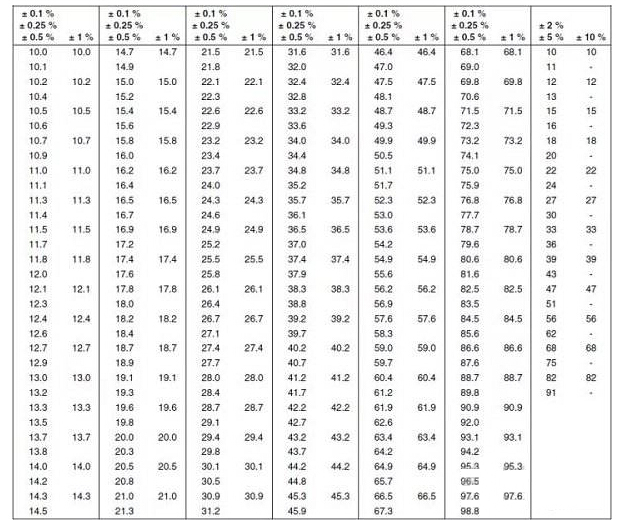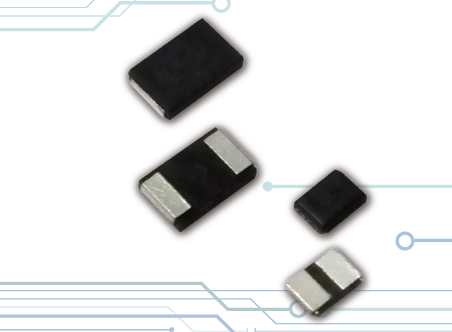Tel:400-803-9333
• Welcome to Microhm

#Position：Home » Industry News

# Precautions for the Use of Resistor Standard Resistance Table

Writer：Microhm Page View：Date：2018-12-17
In the semiconductor industry, there are certain standards for the use of resistors. Generally, the resistance values and models of the relevant resistors will correspond. Resistor manufacturers also use this standard to produce resistors. Each brand of resistor can be found by the resistance standard resistance. Our commonly used resistance standard resistance meters are E24, E96, E12, E192, etc., then we need to pay attention to what matters in the use of these resistance meters, we will carefully analyze.First, the resistance accuracy of different series of resistance meters is different
In the course of use, we must remember that the resistance accuracy of each standard resistance meter is different, that is, the resistance of different precision corresponds to the series of different precision. Usually 10% precision resistors are E12 series, 2% and 5% are E24 series, 1% is E96 series, and 0.1%, 0.25% and 0.5% are E192 series. Just keep in mind the accuracy range of these resistors, you can understand the resistance table we use to query which resistor table.

Second, the numerical significance of the resistor standard resistance table
In each resistor standard resistance series name, the number usually means that the series has several standard resistance values, usually a multiple of 6. In the standard resistance of E12 series resistors, there are 12 different resistance values, and the standard resistance value of E192 series resistors has 192 different resistance values, so we should remember these values in use.

Third, the resistor standard resistance is a geometric series
No matter which type of resistor standard resistance meter we use, we must know that the resistance of each series is almost an equal ratio series. The common ratio is 10 times and the base is 10Ω. For example, the E12 series has a common ratio of 10 to 12 powers, and the E96 series has a common ratio of 10 to 96.So in our use of E24, E96, E12, E192 and other resistance standard resistance table, we can only improve the efficiency of our use by remembering the above three points, we can better query the resistance value we need to use in many projects. It can save a lot of time. However, it is worth noting that the current resistance brand manufacturers also have their own resistance parameter descriptions. Under the relevant conditions, we also need to query the parameters of the relevant brands.